# Lesson 11: Modeling the Spread of Disease

In Lesson 10, we considered a population with a rumor spreading through it. We made the assumption that nobody ever forgot the rumor, and that everyone who didn't hear it had at least some chance to believe it. The model we derived predicted that, under those assumptions, the rumor would eventually spread through the entire population.

Rumors are bad enough, but what if we think about an infectious disease? Under the same basic assumptions (everyone is liable to catch the disease, and once ill a person stays ill), we should expect that, eventually, everyone will become ill.

Luckily for us, most diseases do not obey these assumptions. Let's explore the mathematics of how diseases actually work.

# The Mathematical Model

1. The course of the disease is as follows:
• there is a period of illness, during which the ill person is infectious.
• everyone leaves this infectious stage, and obtains lifelong immunity from the disease. (Call the immune population recovereds.)
2. The period of infectiousness is the same for everyone, and does not vary with time.
3. Anyone who is not immune or currently infectious can catch the disease. (Call these people susceptibles.)
4. The disease is passed through interactions between susceptibles and infectious persons. Recovereds cannot transmit the disease.
5. The chance that a particular interaction will result in a susceptible becoming infectious is the same for everyone, and does not vary with time.
6. The population is constant over time; the susceptible, infectious, and recovered populations change only as described above. (In particular, no new susceptibles are born, and nobody dies of the disease).

These assumptions are more or less true for mild viral diseases like measles and chicken pox. They do not apply, for example, to influenza (which you may catch several times in one season), or to bacterial diseases like cholera.

We want to know how the disease spreads through the population, so we want to keep track of

 variable meaning t time S(t) susceptible population I(t) infected population R(t) recovered population P(t) total population

Let's write down assumption 2 as a differential equation.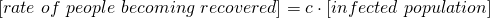where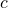is a constant that represents what fraction of the infected population recovers per unit time. We can write this equation symbolically as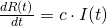Now let's see what influences the susceptible and infected populations. By assumptions 3 and 4, we see that susceptibles can only become infected. So we have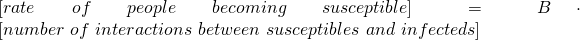where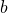represents the chance of a particular interaction resulting in a transmission. Now the number of interactions between susceptibles and infecteds, like we've seen in Lesson 10, should be proportional to the product of the two populations: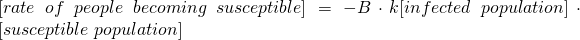Note the minus sign, because the susceptible population is decreasing. Let's call the number. Then, symbolically, we have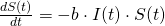Now how does all this affect the rate of change of the infected population? People become infected by losing their susceptible status, and lose their infected status by becoming recovered. As a word equation: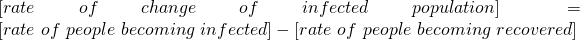We've already worked out each of the two terms on the right-hand side, so we just have to plug them in: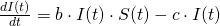We've thus derived what's known as the S-I-R model, or the Kermack-McKendrick model (Kermack and McKendrick were epidemiologists, but the math was discovered by Hilda Phoebe Hudson):# Solving the SIR Model with Maple

How can we understand what the SIR model says about the various subpopulations? From a public-health perspective, we want to make sure that the infected population never gets too large. (If even 10% of the population is sick, that's an impossible strain on public health resources.)

a video walkthrough of this

Observe that our assumption 6 says that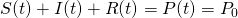That means, if we know two out of the three subpopulations (S, I, R), we can find the third just by subtracting. So we'll focus onand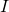.

First, let's see if Maple can solve to find a solution. To do this, tell Maple about the model. We can't use the letter I, so I'll call the susceptible, infected, and recovered populations sus, inf, and rec, respectively.

[> SIR:=diff(sus(t), t) = -b*inf(t)*sus(t), diff(inf(t), t) = b*inf(t)*sus(t)-c*inf(t),diff(rec(t),t)=c*inf(t)Let's pick some values ofandfor the sake of definiteness, say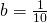and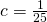:

And then we'll try to solve the initial value problem assuming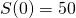and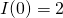and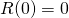:

[> dsolve({SIR,sus(0)=50,inf(0)=2,rec(0)=0})What happened here? It turns out that the differential equation just doesn't have a nice solution. Maple did its best, but that wasn't really good enough.

Maple still has a trick up its sleeve, though. We will invoke dsolve with the option numeric, and tell Maple to keep track for the first 10 days:

[> solution:=dsolve({SIR,sus(0)=50,inf(0)=2,rec(0)=0},numeric)

This doesn't give us an answer, but it stores the solution attempt in a variable called solution. Now let's plot that. We need the plots library:which includes the command odeplot:

[> odeplot(solution, [[t, sus(t), color = blue], [t, inf(t), color = red]], 0 .. 10)

odeplot asks for a dsolve, the part of the solution we'd like it to plot, and a range of t values for which we'd like to have the solution plotted. Here I've asked it to plotand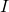for the first 10 days of the outbreak, with the susceptible population in blue and the infected population in red.

Looking at the graph, we see that by day 2 of the outbreak, almost everyone is infected.If we change the parameters to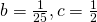, we see that the peak of the outbreak occurs later -- on day 3 -- but is a lot lower. Play around with some values ofandand see what effect they have on the peak and duration of the outbreak.Let's take a longer view, by resetting the range to cover the first 30 days of the outbreak. We can see that, although the number of infected persons has dropped nearly to zero, there is still a stable population of susceptibles:We can also plot the number of recovereds on the same plot:# Some Other Assumptions

Immunity to a virus doesn't actually last forever. So we might consider updating the model to indicate that, as time goes on, some fractionof the recovered population stops being immune: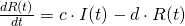and becomes susceptible again: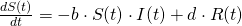Let's enter this in Maple:and pick a value for the loss-of-immunity rate (say it's low compared to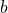and, maybe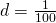), have Maple solve and plot. During the initial outbreak, this doesn't change much:but as time goes on, it results in flare-ups of the disease every so often, as well as a small stable reservoir of infected individuals, and a significant reservoir of susceptible individualsObserve that the flareups get less severe over time. It's fun to play around with the values of,, and, to see how they impact the frequency and severity of spikes in the infected and susceptible populations.

Some other things we could take into account:

• The virulence of the disease: does it actually cause people to die? How quickly?
• If we allow the total population to vary over time, do the susceptible, infected, and recovered populations all have the same natural reproductive rate?
• Are new births automatically susceptible? Are births to infected persons automatically infected? Perhaps there is only a chance of a birth to an infected or recovered mother, that the mother passes on either the disease or the immunity.

Each of these situations will contribute a term to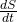,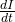,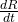, or perhaps all three, which we can then use dsolve and odeplot to explore the effect of.